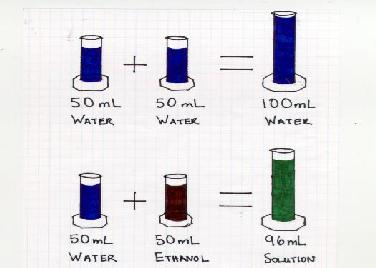Miscibility of Fluids

Miscibility of FluidsThe students were asked, "what is a fluid?" (A fluid is defined in the theory section) Once a fluid was adequately defined, the students were asked to predict what would happen if 50mL of water was mixed with another 50mL of water. We then mixed two graduated cylinders, each containing 50mL of water into a 100mL graduated cylinder. The result was 100mL of water total.

The students were then asked what would happen if 50mL of water and 50mL of ethanol were mixed. We again mixed 50mL of water with 50mL of ethanol. The result was a total volume of approximately 96mL. We asked the students to explain this. No student, out of the 350 asked, could explain what had happened. Rather than give the answer away, we presented another demonstration.

We took 500mL of rice and mixed it with another 500mL of rice. We explained to the students that the rice represented the water molecules. The result was 1000mL of rice. We then took 500mL of rice and 500mL of rigatoni noodles and mixed them. The rigatoni noodles represented the ethanol. The result was approximately 650mL to 700mL. The students' eyes all lit up with understanding by the end of this demo.

Explanation for teacher: The molecules of the ethanol are smaller than the water molecules, allowing the ethanol molecules to fall between the water molecules.